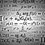Fallacy-explain the point of fallacy

Let a=b so a^2 = ab or a^2-b^2 = ab-b^2 or (a+b)(a-b) = b(a-b) or a+b = b

now, putting the value of a, b+b=b or 2b=b or 2=1 :P But how??Note by Shubham Sharma
6 years, 5 months ago

This discussion board is a place to discuss our Daily Challenges and the math and science related to those challenges. Explanations are more than just a solution — they should explain the steps and thinking strategies that you used to obtain the solution. Comments should further the discussion of math and science.

When posting on Brilliant:

• Use the emojis to react to an explanation, whether you're congratulating a job well done , or just really confused .
• Ask specific questions about the challenge or the steps in somebody's explanation. Well-posed questions can add a lot to the discussion, but posting "I don't understand!" doesn't help anyone.
• Try to contribute something new to the discussion, whether it is an extension, generalization or other idea related to the challenge.
• Stay on topic — we're all here to learn more about math and science, not to hear about your favorite get-rich-quick scheme or current world events.

MarkdownAppears as
*italics* or _italics_ italics
**bold** or __bold__ bold

- bulleted
- list

• bulleted
• list

1. numbered
2. list

1. numbered
2. list
Note: you must add a full line of space before and after lists for them to show up correctly
paragraph 1

paragraph 2

paragraph 1

paragraph 2

> This is a quote
This is a quote
# I indented these lines
# 4 spaces, and now they show
# up as a code block.

print "hello world"
# I indented these lines
# 4 spaces, and now they show
# up as a code block.

print "hello world"
MathAppears as
Remember to wrap math in $$...$$ or $...$ to ensure proper formatting.
2 \times 3 $2 \times 3$
2^{34} $2^{34}$
a_{i-1} $a_{i-1}$
\frac{2}{3} $\frac{2}{3}$
\sqrt{2} $\sqrt{2}$
\sum_{i=1}^3 $\sum_{i=1}^3$
\sin \theta $\sin \theta$
\boxed{123} $\boxed{123}$

Sort by:

Since a-b=0, in the 4th step you have divided 0 by 0. In mathematics division by zero is meaningless.

Fallacy is an argument built on the base of poor reasoning. Your conclusion might be true or maybe not but it is still fallacious.

- 6 years, 5 months ago

yeah, i also got it on wikipedia...

- 6 years, 5 months ago

Instead of a=b, let's let a-b=k

$a=b+k$

$a^2 = ab+ak$

$a^2-b^2 = ab+ak-b^2$

$(a+b)(a-b) = b(a-b)+ak$

$a+b=b+\frac{ak}{a-b}$

You are saying that $lim_{x \rightarrow 0} \frac{a \times x}{x} = 0$, which causes this fallacy.

- 6 years, 5 months ago

dude dats a bit hi-fi!! limit? dats out of range :D

- 6 years, 5 months ago

We are seeing what happens if b is close to a, to determine what went wrong.

Likewise, you cannot evaluate $\frac{x-4}{\sqrt{x}-2}$ where x=4 directly by substitution, although it is possible to evaluate it. By substitution, we get $\frac{0}{0}$ which is kind of meaningless.

- 6 years, 5 months ago

yup..

- 6 years, 5 months ago

That's not meaningless. That is everything. What times 0 equals 0? is what that's asking.

- 5 years, 7 months ago

I agree that $\frac{0}{0}$ can be any number, but the fact that it reduces to $\frac{0}{0}$ says nothing more about the value an expression is unless we know that the $0$s mean.

- 5 years ago

You just can't cut (a-b) as 0/0 is indeterminate.

- 5 years ago

a=b means a-b=0, and division by 0 is meaningless.

- 4 years, 3 months ago